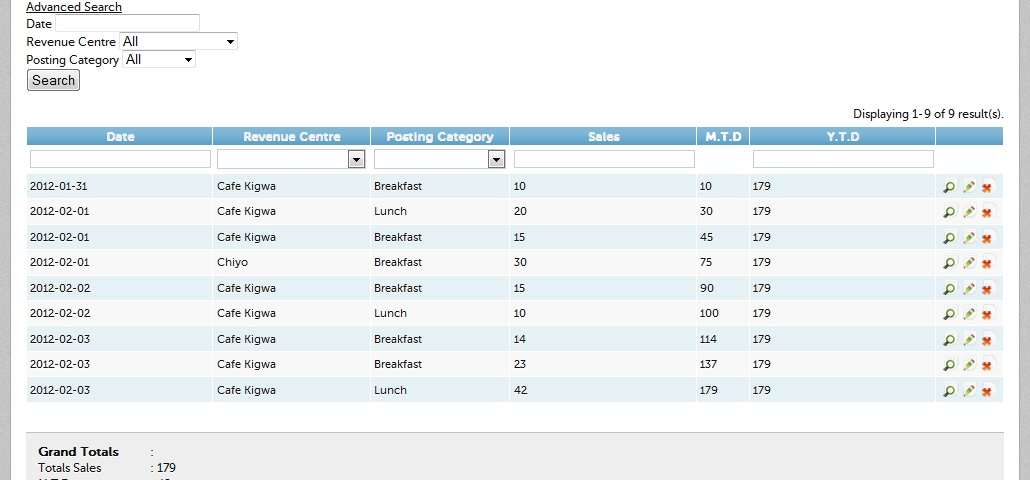# CGridView computations

I’m using CGridView to display data for a report i.e in the admin view

<?php \$this->widget(‘zii.widgets.grid.CGridView’, array(

``````'id' =&gt; 'ccp-grid',

'dataProvider' =&gt; &#036;model-&gt;search(),

'filter' =&gt; &#036;model,

'columns' =&gt; array(

//'id',

'date',

array(

'name'=&gt;'rvc_id',

'value'=&gt;'GxHtml::valueEx(&#036;data-&gt;rvc)',

'filter'=&gt;GxHtml::listDataEx(Rvc::model()-&gt;findAllAttributes(null, true)),

),

array(

'name'=&gt;'postingcat_id',

'value'=&gt;'GxHtml::valueEx(&#036;data-&gt;postingcat)',

'filter'=&gt;GxHtml::listDataEx(Postingcat::model()-&gt;findAllAttributes(null, true)),

),

'sales',

'mtd',

.......
``````

I have a mysql table

INSERT INTO `ccp` (`id`, `date`, `rvc_id`, `postingcat_id`, `sales`,‘mtd’) VALUES

(

1 2012-02-01 1 1 10 10

2 2012-02-01 1 2 20 20

3 2012-02-01 2 1 5 5

4 2012-02-01 2 2 15 15

5 2012-02-02 1 1 20 30

6 2012-02-02 1 2 10 30

7 2012-02-02 2 2 20 35

);

nb: I have calculated the values of mtd above manually for explanation purpose.

I need to calculate the monthly total to date(mtd) of each posting category(postingcat_id) per revenue centre(rvc_id) and display in mtd column

• posting categories e.g breakfast,lunch,supper
• revenue center e.g cafe 1, la pizerria

mtd is the sum of all sales of a given posting category in a revenue centre in a given month to date

Maybe this extension helps.

An inefficient but straightforward way of doing it would be to add a getMTD function to your ccp model that would calculate the MTD for that row of the table (obviously you’d have to hit the database once for each row you display). You could then reference it in the CGridView.

If you need more efficiency, you could switch over to using an SQLDataProvider to feed your CGridView and write sql to calculate the MTD and return it as one of your columns.

Thanks Jobla and Zilles for you help.much appreciation. I worked on zilles idea and am still looking at jobla’s. I created a class

<?php

Yii::import(‘zii.widgets.grid.CGridColumn’);

class getMTD extends CGridColumn {

``````private &#036;_mtd = 0;

public function renderDataCellContent(&#036;row, &#036;data) { // &#036;row number is ignored

&#036;this-&gt;_mtd += &#036;data-&gt;sales;

echo &#036;this-&gt;_mtd;

}
``````

}

array(

``````'name'=&gt;'mtd',

'value'=&gt;&#036;model-&gt;getMTD(),

),
``````

It calculates the monthly totals however I can’t figute out how to calculate the monthy totals in a given revenue centre per posting category. Please help. Thanks in advance

2517You shouldn’t have to extend CGridColumn.

If you add getMTD() to your Ccp model, then you can just reference "MTD" in the column list.

I was thinking you could use MySql to calculate the mtd value.

Something like this: (untested code)

``````

function getMTD()

{

\$conn=Yii::app()->db;

\$command=\$conn->createCommand( 'SELECT SUM(sales) FROM ccp

WHERE MONTH(DATE)=:month AND YEAR(DATE)=:year AND

rvc_id=:rvc AND postingcat_id=:pc AND id<=:id');

\$data = \$command->queryScalar(

array(

'month'=>date('m',\$this->date),

'year'=>date('Y',\$this->date),

'rvc'=>\$this->rvc_id,

'pc'=>\$this->postingcat_id,

'id'=>\$this->id

));

return \$data;

}

``````

This code assumes that orders are placed in the table in chronological order, so you will have to adjust it if that’s not a safe assumption.

Alternatively, you can work this sql into a query:

``````
SELECT *, (SELECT SUM(sales) FROM ccp t2

WHERE MONTH(t1.date)=MONTH(t2.date) AND YEAR(t1.date)=YEAR(t2.date) AND t1.rvc_id=t2.rvc_id AND

t1.postingcat_id=t2.postingcat_id AND t2.id<=t1.id) AS mtd FROM ccp t1

``````

And populate your CGridView with a SQLDataProvider

Thanks zilles for the help.

I tried this but it returns nothing.

``````
function getMTD()

{

\$conn=Yii::app()->db;

\$command=\$conn->createCommand( 'SELECT SUM(sales) FROM ccp

WHERE MONTH(DATE)=:month AND YEAR(DATE)=:year AND

rvc_id=:rvc AND postingcat_id=:pc AND id<=:id');

\$data = \$command->queryScalar(

array(

'month'=>date('m',\$this->date),

'year'=>date('Y',\$this->date),

'rvc'=>\$this->rvc_id,

'pc'=>\$this->postingcat_id,

'id'=>\$this->id

));

return \$data;

}

``````

When I run this query

``````
SELECT *, (SELECT SUM(sales) FROM ccp t2

WHERE MONTH(t1.date)=MONTH(t2.date) AND YEAR(t1.date)=YEAR(t2.date) AND t1.rvc_id=t2.rvc_id AND

t1.postingcat_id=t2.postingcat_id AND t2.id<=t1.id) AS mtd FROM ccp t1

``````

in mysql it gives me the right results but I still cant figure out how to populate those results in cgridview.

I did

``````
function getMTD()

{

\$sql= 'SELECT ( SELECT SUM( sales )FROM `ccp` `t`

WHERE MONTH( t1.date ) = MONTH( t.date )

AND YEAR( t1.date ) = YEAR( t.date )

AND t1.rvc_id = t.rvc_id

AND t1.postingcat_id = t.postingcat_id

AND t.id <= t1.id ) as mtd from ccp t1';

\$conn=Yii::app()->db;

\$command=\$conn->createCommand(\$sql);

\$data = \$command->queryscalar();

return \$data;

}

``````

Yeah, I don’t have enough information to write code that’s definitely going to work for you. I was just trying to get you on the right track. It should be close though. You might try debugging this. I don’t know how you’re storing dates in the object. If they’re strings then you might just need to parse them before pulling out the components.

``````

'month'=>date('m',strtotime(\$this->date)),

'year'=>date('Y',strtotime(\$this->date)),

``````

But in general, try and figure out what the code is trying to do and fill in the missing pieces.

Yeah, you can’t use it this way. Read up on SQLDataProvider to see how you would use this sql query with a CGridView.

You might take a look at what this guy did:

http://www.yiiframework.com/forum/index.php?/topic/28597-sum-if/

He seems to have had a similar issue to you and solved it nicely.

Thanks for your guidance Zilles.Much appreciation.It worked.This has been a great learning experience.For those wanting to use DAO instead of AR:

Create a function in your model:

``````
public \$mtd;

public \$id;

public function getMtd()

{

\$sql = 'SELECT *,(SELECT SUM(sales) FROM tbl_ccp t2

WHERE MONTH(t1.date)=MONTH(t2.date) AND YEAR(t1.date)=YEAR(t2.date) AND t1.rvc_id=t2.rvc_id

AND t1.postingcat_id=t2.postingcat_id AND t2.id<=t1.id) AS mtd

FROM tbl_ccp t1, tbl_rvc, tbl_postingcat

WHERE rvc_id = tbl_rvc.id

AND postingcat_id = tbl_postingcat.id

GROUP BY DATE, rvc_id, postingcat_id';

\$sql_count = 'SELECT COUNT(id) FROM tbl_ccp';

return new CSqlDataProvider(\$sql, array(

'totalItemCount' => Yii::app()->db->createCommand(\$sql_count)->queryScalar(),

'sort' => array(

'attributes' => array(

'date',

),

),

'pagination' => array(

'pageSize' => 15,

),

));

}

``````

``````
<?php \$this->widget('zii.widgets.grid.CGridView', array(

'dataProvider' => \$model->getMtd(),

'columns' => array(

array(

'name'=>'Category',

'value'=>'\$data["postingcat_name"]', //if you declared in relationship it will show name instead of Id.

),

'mtd::M.T.D',

),

));

?>

``````

If you put your function in the controller accress with \$this->getMtd()

If in model use \$model->getMtd()

If you want a summary total at the footer:

1. create a funtion to calculate the totals.

2. In the columns

``````
array(